# Water overflow

A rectangular container that has a length of 30 cm, a width of 20 cm, and a height of 24 cm is filled with water to a depth of 15 cm. When an additional 6.5 liters of water are poured into the container, some water overflows. How many liters of water overflow the container?

x =  1.1 l

### Step-by-step explanation: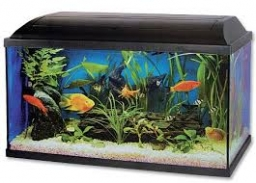Did you find an error or inaccuracy? Feel free to write us. Thank you!Tips to related online calculators
Do you know the volume and unit volume, and want to convert volume units?

## Related math problems and questions:

• AquariumHow many liters of water can fit in an aquarium measuring 30, 15 and 20 cm?
• Reservoir 3How many cubic feet of water is stored in the reservoir that has a length of 200 feet, a width of 100 feet, an overflow depth of 32 feet, and a current water level of 24 feet?
• Water levelThe glass container has a cuboid shape with dimensions of the bottom 24 cm and 12 cm. The height of the water is 26 cm. Calculate the body's volume, which is sunk into the water if the water level rise by 3 cm.
• For thinkingsThe glass cube dive into the aquarium, which has a length of 25 cm, width 20 cm and height of 30 cm. Aquarium water rises by 2 cm. a) What is the volume of a cube? b) How many centimeters measure its edge?
• Liters in cylinderDetermine the height at which level 24 liters of water in a cylindrical container having a bottom diameter 36 cm.
• Cuboid aquariumCuboid 25 times 30 cm. How long is third side if cuboid contains 30 liters of water?
• The cuboidThe cuboid is full to the brim with water. The external dimensions are 95 cm, 120 cm, and 60 cm. The thickness of all walls and the bottom is 5 cm. How many liters of water fit into the cuboid?
• Water in aquariumThe aquarium cuboid shape with a length of 25 cm and a width of 30 cm is 9 liters of water. Calculate the areas which are wetted with water.
• Bricks wallThere are 5000 bricks. How high wall thickness of 20 cm around the area which has dimensions 20 m and 15 m can use these bricks to build? Brick dimensions are 30 cm, 20 cm and 10 cm.
• Water blockA block with a 50 cm2 base is filled with water 5 cm under the edge. How many can sugar cubes with 2 cm edge be thrown into a container that overflow water?
• Pool coating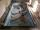How many tiles 25 cm × 15 cm need to coat the bottom and sidewalls of the pool with bottom dimensions 30 m × 5 m, if the pool can fit up to 271500 liters of water?
• Snails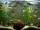How many liters of water will fit in an aquarium with bottom dimensions of 30 cm and 25 cm and a height of 60 cm, if we pour water up to a height of 58 cm? How many most snails can we keep in an aquarium, if we know that snails need 600 cm ^ 3 of water fo
• Workers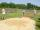Workers digging a jump pit in the schoolyard. The pit has a cuboid shape with a length 12 m, a width of 20 dm, and a depth of 36 cm. They excavate 0.4 cubic meters of soil an hour. How much time (hours and minutes) is need to excavate this pit?
• ConvaHow many liters of water fit into the shape of a cylinder with a bottom diameter 20 cm and a height 45 cm?
• AquariumThe box-shaped aquarium is 40 cm high; the bottom has dimensions of 70 cm and 50 cm. Simon wanted to create an exciting environment for the fish, so he fixed three pillars to the bottom. They all have the shape of a cuboid with a square base. The base edg
• Stone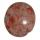When Peter threw stone in a box of water he discovered that the water level has risen by 6 cm. The box has a cuboid shape, the bottom has dimensions of 24 cm and 14 cm, height is 40 cm. What volume has a stone?
• Cube-shaped containerThe cube-shaped container has a height of 52 cm and a square base. The container was filled to the brim with water, then we immersed a metal cube in it, which caused 2.7 l of water to flow out of the container. After removing the cube from the water, the